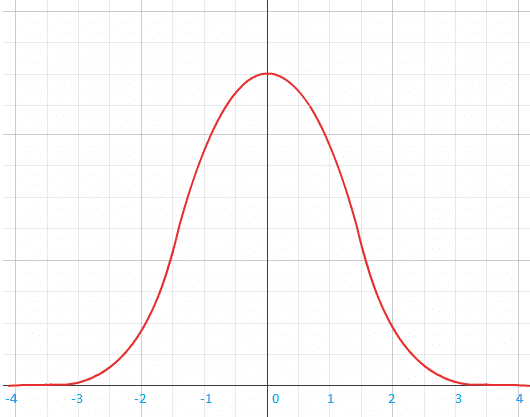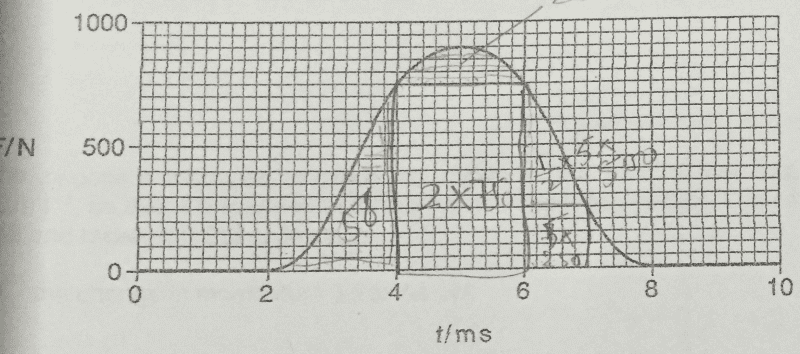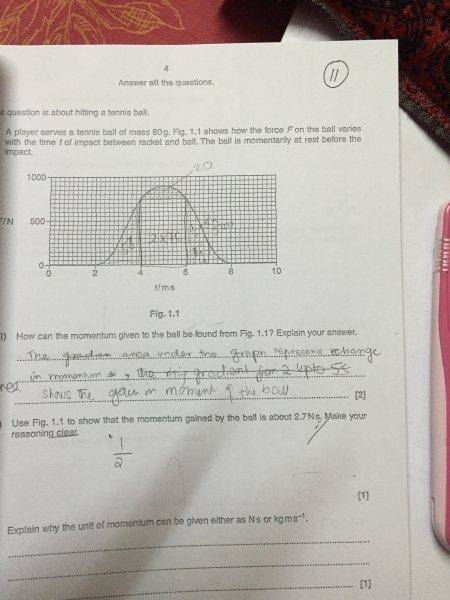# Mean value of graph - is my reasoning correct?

• PhysicStud01
If you are given that the curve is gaussian with standard deviation 1 sec., you can find the area under the curve by taking the square of the standard deviation.f

## Homework Statement

Consider a gaussian graphlet 5 quares on y -axis represent 1units

## Homework Equations

is it correct for me to say that the average value of the quantity on the y-axis is given by
y_av = (y_min + y_max) / 2
where y_min is smallest value of y in the graph = 0
y_max is the maximum value of y in graph = 2.4
average value of y, y_av = (0 + 2.4) /2 = 1.2

## The Attempt at a Solution

then, to find the area under graph, i use
area = y_av * (3 - -3) = y_av * 6 = 7.2

is this correct or wrong. in the above, i have all the things that i would if this was a question.

am i missing an assumption. the answer seems correct but is the method correct.
note that at this a-level, i don't have to know equation of gaussian graph nor do i do integration.

is this the correct way to find area under graph for non-linear graphs?? is there a general formula (not integration) for lower levels?

let 5 quares on y -axis represent 1units
Try it again, and pay attention to details.

It isn't clear what you mean by "The average value of the quantity on the y-axis". You should state the problem exactly as your course materials state it.

Try it again, and pay attention to details.
oh, it's 4 squares. any way this was only an example. let's say its 5square. i don't have the correct graph. and take the values i gave as the correct value
It isn't clear what you mean by "The average value of the quantity on the y-axis". You should state the problem exactly as your course materials state it.
it's not really a coruse materials.

actuallly, y-axis is Force and x-axis is time. i need to find change in momentum. the initial momentum is zero.
do i really need to count sqaures or is there an easier way to do it

i don't have the correct graph. and take the values i gave as the correct value
When asking for help, please don't give us a picture of a pineapple and call it a carrot.
do i really need to count sqaures or is there an easier way to do it
There are easier ways, however, you have already stated the following ---
note that at this a-level, i don't have to know equation of gaussian graph nor do i do integration.
--- and that pretty much sticks you with "counting squares."

i need to find change in momentum. the initial momentum is zero.
Do you mean that the momentum of some mass is 0 at x = 0 and that y represents the net applied force on the mass as a function of time ?

What do you mean by "the change in momentum"? Change between what time and what other time?

Do you mean that the momentum of some mass is 0 at x = 0 and that y represents the net applied force on the mass as a function of time ?

What do you mean by "the change in momentum"? Change between what time and what other time?
momentum is zero for x-axis < -3.
x-axis would be time. of course time cannot be zero. so, let's just assume.

the graph would actually be that of a tennis ball.
When asking for help, please don't give us a picture of a pineapple and call it a carrot.

There are easier ways, however, you have already stated the following ---

--- and that pretty much sticks you with "counting squares."
so, what about the method i first explained. about average force for the time involved. (the time for which the force is not zero)

momentum is zero for x-axis < -3.
x-axis would be time. of course time cannot be zero. so, let's just assume.

the graph would actually be that of a tennis ball.

It isn't clear what you are talking about. I suggest you write an accurate and complete description of the problem you are trying to solve.

so, what about the method i first explained. about average force for the time involved.
That's "counting squares."need to show that momentum gained by ball is 2.7Ns

That's "counting squares."
it's not counting squares, it's taking the average value of the force

View attachment 77175
need to show that momentum gained by ball is 2.7Ns

That isn't a complete statement of a problem.

it's not counting squares, it's taking the average value of the force

To find the average force acting between two times, say x = 4 and x = 6 seconds, you can divide the area of the graph under the curve between x = 4 and x = 6 seconds by the length (6-4) = 2. So counting the squares can be one step of that process.

.If you are given that the curve is gaussian with standard deviation 1 sec. , you can find the area by using a table of the normal distribution.

That isn't a complete statement of a problem.

To find the average force acting between two times, say x = 4 and x = 6 seconds, you can divide the area of the graph under the curve between x = 4 and x = 6 seconds by the length (6-4) = 2. So counting the squares can be one step of that process.

.If you are given that the curve is gaussian with standard deviation 1 sec. , you can find the area by using a table of the normal distribution.
so, the way i calculate the average value is wrong? even if i obtain the answer + by counting squares, i can't arrive at the answer

so, the way i calculate the average value is wrong? even if i obtain the answer + by counting squares, i can't arrive at the answer

If you refuse to state the problem in a coherent manner, nobody actually knows whether what you are doing is correct.

If you refuse to state the problem in a coherent manner, nobody actually knows whether what you are doing is correct.
here's the questionYou need the integral of the F(t) curve between 2 ms and 8 ms. Do it by counting the small squares enclosed, and taking into account how much momentum one square means.

You have mentioned finding the average value of force. If you were taught to do the the problem this way, you could add up the force at intervals corresponding to the vertical lines on the graph, for example at times .002 sec,.0022 sec, .00024 sec ...0078 sec .008 sec and divide this sum by the number of terms you added. The average force times the length of the interval from .002 sec to .008 gives you an approximation for the area under the curve.

As echild says, you can also find the area under the curve by counting squares.